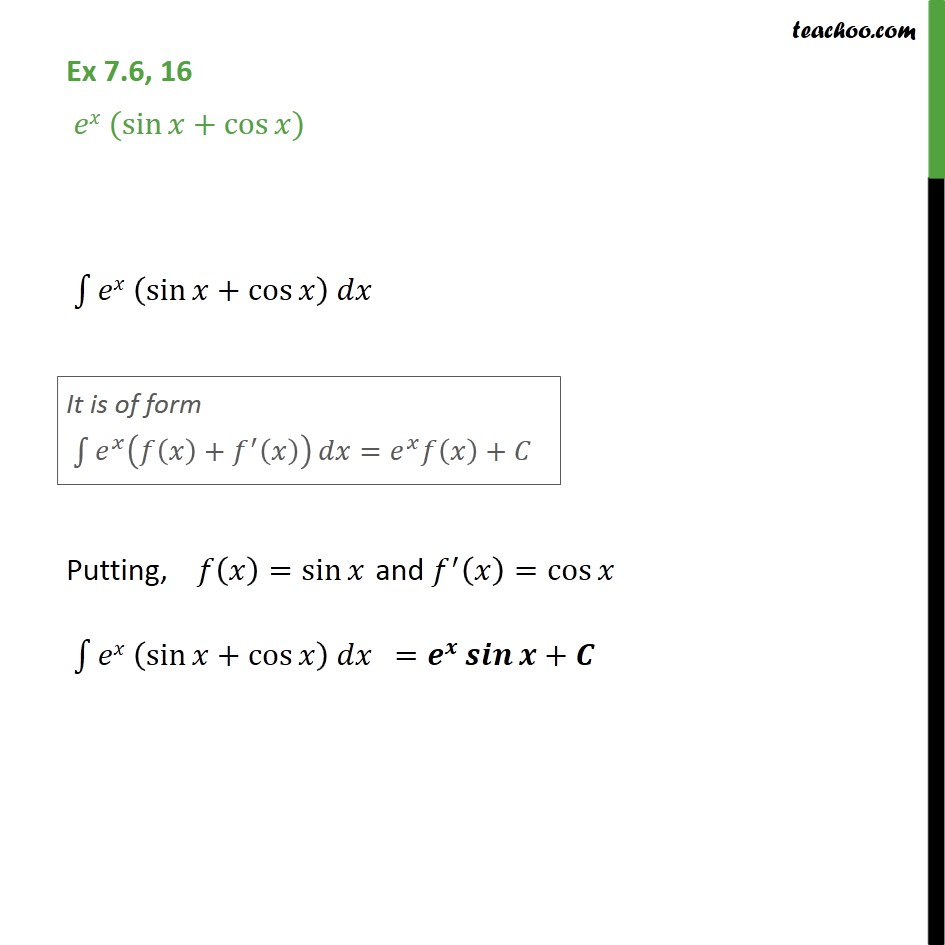Ex 7.6

Chapter 7 Class 12 Integrals
Serial order wiseLearn in your speed, with individual attention - Teachoo Maths 1-on-1 Class

### Transcript

Ex 7.6, 16 - Chapter 7 Class 12 Integrals - NCERT e^x (sin x + cos x) ∫e^x (sin x + cos x) It is of form ∫ e^x (f(x) + f'(x)) dx = e^x f(x) + C Putting, f(x) = sin x, f'(x) = cos x ∫e^x (sin x + cos x) = e^x sin x + C